Back to the main Index

# Phonons and Born effective charges with Abinit and AbiPy¶

This lesson discusses how to compute phonon band structures, DOS and Born effective charges with Abinit and AbiPy. The discussion closely follows the second lesson on DFPT available on the Abinit web site. More specifically, we will discuss how to

• Perform a convergence study for the phonon frequencies at $\Gamma$ as function of ecut
• Compute the full phonon band structure of AlAs with the inclusion of LO-TO splitting
• Obtain thermodynamic properties within the harmonic approximation

We assume that you have read the references mentioned in the first Abinit lesson on DFPT. You might find additional material, related to the present section, in the following references:

If you are already familiar with python and AbiPy-Abinit are already installed and configured, you may want to use directly the command line interface. See the README.md file in the directory of this lesson explaining how to analyze the data from the shell using ipython and matplotlib.

## Phonon frequencies at $\Gamma$ as function of ecut¶

Before starting, we need to import the python modules and the functions we will need in the notebook:

In :
# Use this at the beginning of your script so that your code will be compatible with python3
from __future__ import print_function, division, unicode_literals

import numpy as np
import warnings
warnings.filterwarnings("ignore") # to get rid of deprecation warnings

from abipy import abilab
abilab.enable_notebook() # This line tells AbiPy we are running inside a notebook
import abipy.flowtk as flowtk

# This line configures matplotlib to show figures embedded in the notebook.
# Replace inline with notebook in classic notebook
%matplotlib inline

# Option available in jupyterlab. See https://github.com/matplotlib/jupyter-matplotlib
#%matplotlib widget


and an useful function from the lesson_dfpt module that will be used to generate our DFPT flows:

In :
from lesson_dfpt import make_scf_input
abilab.print_source(make_scf_input)

Out:
def make_scf_input(ecut=2, ngkpt=(4, 4, 4)):
"""
This function constructs an AbinitInput to perform a GS-SCF calculation in crystalline AlAs.

Args:
ecut: cutoff energy in Ha.
ngkpt: 3 integers specifying the k-mesh for the electrons.

Return:
AbinitInput object
"""
# Initialize the AlAs structure from an internal database. Use the pseudos shipped with AbiPy.
gs_inp = abilab.AbinitInput(structure=abidata.structure_from_ucell("AlAs"),
pseudos=abidata.pseudos("13al.981214.fhi", "33as.pspnc"))

# Set the value of the Abinit variables needed for GS runs.
gs_inp.set_vars(
nband=4,
ecut=ecut,
ngkpt=ngkpt,
nshiftk=4,
shiftk=[0.0, 0.0, 0.5,   # This gives the usual fcc Monkhorst-Pack grid
0.0, 0.5, 0.0,
0.5, 0.0, 0.0,
0.5, 0.5, 0.5],
ixc=1,
nstep=25,
diemac=9.0,
tolvrs=1.0e-10,
#iomode=3,
)

return gs_inp


The function makes some assumptions for important parameters such as the crystalline structure and the pseudos. This is done on purpose to keep the code as simple as possible. It should not be so difficult to generalize the implementation to take into account other cases. Let's start to play with our new function:

In :
scf_input = make_scf_input()
scf_input

Out:
############################################################################################
# SECTION: basic
############################################################################################
nband 4
ecut 2
ngkpt 4 4 4
nshiftk 4
shiftk
0.0 0.0 0.5
0.0 0.5 0.0
0.5 0.0 0.0
0.5 0.5 0.5
ixc 1
nstep 25
tolvrs 1e-10
############################################################################################
# SECTION: gstate
############################################################################################
diemac 9.0
############################################################################################
# STRUCTURE
############################################################################################
natom 2
ntypat 2
typat 1 2
znucl 13 33
xred
0.0000000000 0.0000000000 0.0000000000
0.2500000000 0.2500000000 0.2500000000
acell 1.0 1.0 1.0
rprim
0.0000000000 5.3050000000 5.3050000000
5.3050000000 0.0000000000 5.3050000000
5.3050000000 5.3050000000 0.0000000000
In :
print(scf_input.structure)

Full Formula (Al1 As1)
Reduced Formula: AlAs
abc   :   3.970101   3.970101   3.970101
angles:  60.000000  60.000000  60.000000
Sites (2)
#  SP       a     b     c
---  ----  ----  ----  ----
0  Al    0     0     0
1  As    0.25  0.25  0.25

In :
scf_input.structure.plot();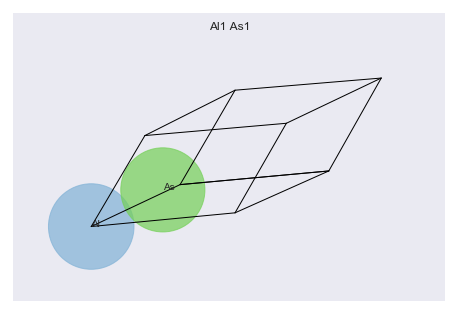We are using the same pseudopotentials than the official tutorial. Note that the xc functional is LDA in both pseudos but with a different parametrization. This is the reason why we are using ixc in the input file.

In :
for pseudo in scf_input.pseudos:
print(pseudo, "\n")

<NcAbinitPseudo: 13al.981214.fhi>
summary: Aluminum, fhi98PP : Hamann-type, LDA CA PerdewWang, l=2 local
number of valence electrons: 3.0
maximum angular momentum: d
angular momentum for local part: d
XC correlation: PW
supports spin-orbit: False
radius for non-linear core correction: 0.0
hint for low accuracy: ecut: 0.0, pawecutdg: 0.0
hint for normal accuracy: ecut: 0.0, pawecutdg: 0.0
hint for high accuracy: ecut: 0.0, pawecutdg: 0.0

<NcAbinitPseudo: 33as.pspnc>
summary: Troullier-Martins psp for element  As        Thu Oct 27 17:37:14 EDT 1994
number of valence electrons: 5.0
maximum angular momentum: p
angular momentum for local part: p
XC correlation: LDA_XC_TETER93
supports spin-orbit: False
radius for non-linear core correction: 2.0573171556401
hint for low accuracy: ecut: 0.0, pawecutdg: 0.0
hint for normal accuracy: ecut: 0.0, pawecutdg: 0.0
hint for high accuracy: ecut: 0.0, pawecutdg: 0.0



As you can see, we essentialy have a standard input to perform a GS calculation. This object will represent the building block for our DFPT calculation with AbiPy.

It this is not your first time you use the DFPT part of Abinit, you already know that phonon calculations require an initial GS run to produce the WFK file followed by a DFPT run that reads the WFK file and solves the Sternheimer equations for $N_{\text{irred}}(q)$ atomic perturbations where $N_{\text{irred}}$ is the number of independent atomic displacements (assuming $q$ belongs to the k-mesh).

If you try to do a convergence study wrt ecut without multi-datasets, you will likely start from an initial GS input file with a given value of ecut, use it as a template to generate the DFPT input files, create symbolic links to the WFK file produced in the first GS step and then instruct Abinit to read this file with irdwfk. Once you have a set of input files that work for a particular ecut, one can simply replicate the set of directories and files and use a script to change the value of ecut in the input files. Then, of course, one has to run the calculations manually, collect the results and produce nice plots to understand what is happening.

This approach is obviously boring and error-prone if you are a human being but it is easy to implement in an algorithm and machines do not complain if they have a lot of repetive work to do! There are also several technical advantages in using this task-based approach vs multi-datasets but we discuss this point in more details afterwards.

If the machine could speak, it will tell you: give me an object that represents an input for GS calculations, give me the list of q-points you want to compute as well as the parameters that must be changed in the initial input and I will generate a Flow for DFPT calculations. This logic appears so frequenty that we decided to encapsulate it in the flowtk.phonon_conv_flow factory function:

In :
from lesson_dfpt import build_flow_alas_ecut_conv
abilab.print_source(build_flow_alas_ecut_conv)

Out:
def build_flow_alas_ecut_conv(options):
"""
Build and return Flow for convergence study of phonon frequencies at Gamma as function of ecut.
"""
scf_input = make_scf_input()
return flowtk.phonon_conv_flow("flow_alas_ecut_conv", scf_input, qpoints=(0, 0, 0),
params=["ecut", [4, 6, 8]])


Let's call the function to build our flow:

In :
flow = build_flow_alas_ecut_conv(options=None)

flow.show_info()

<Flow, node_id=345794, workdir=flow_alas_ecut_conv>
Number of works: 6, total number of tasks: 9
Number of tasks with a given class:

------------  --------
PhononTask           6

and call the get_graphviz method to visualize the connection among the Tasks:

In :
flow.get_graphviz()

Out:
In :
# matplotlib version based on networkx
#flow.plot_networkx(with_edge_labels=True);


The flow contains three independent groups of tasks, one group per each value of ecut specified in params.

In :
for work in flow:

w0_t0 uses ecut: 4
w1_t0 uses ecut: 4
w1_t1 uses ecut: 4
w2_t0 uses ecut: 6
w3_t0 uses ecut: 6
w3_t1 uses ecut: 6
w4_t0 uses ecut: 8
w5_t0 uses ecut: 8
w5_t1 uses ecut: 8

In :
flow.get_vars_dataframe("ecut")

Out:
ecut class

Each group represents a Workflow and consists of one ScfTask(red circle) that solves the KS equations self-consistently producing a WFK file that will be used by the two children (PhononTasks - blue circles) to compute the first-order change of the wavefunctions due to one of the irreducible atomic pertubations.

Note that phonon_conv_flow invokes Abinit under the hood to get the list of irreducible perturbations and uses this information to build the flow. This explains why we have two PhononTasks per $q$-point instead of the total number of phonon modes that equals $3*N_{atom}=6$.

Perhaps a table with the values of the input variables associated to the DFPT perturbation will help. None means that the variable is not defined in that particular input.

In :
flow.get_vars_dataframe("rfphon", "rfatpol", "rfdir", "qpt", "kptopt")

Out:
rfphon rfatpol rfdir qpt kptopt class
w0_t0 None None None None None ScfTask
w1_t0 1 [1, 1] [1, 0, 0] [0.0, 0.0, 0.0] 2 PhononTask
w1_t1 1 [2, 2] [1, 0, 0] [0.0, 0.0, 0.0] 2 PhononTask
w2_t0 None None None None None ScfTask
w3_t0 1 [1, 1] [1, 0, 0] [0.0, 0.0, 0.0] 2 PhononTask
w3_t1 1 [2, 2] [1, 0, 0] [0.0, 0.0, 0.0] 2 PhononTask
w4_t0 None None None None None ScfTask
w5_t0 1 [1, 1] [1, 0, 0] [0.0, 0.0, 0.0] 2 PhononTask
w5_t1 1 [2, 2] [1, 0, 0] [0.0, 0.0, 0.0] 2 PhononTask

If the meaning of these variables is not clear, you can consult the Abinit documentation e.g. the documentation of the rfatpol input variable or access the documentation directly from python with:

In :
abilab.docvar("rfatpol")

Out:

[1, 1]

## Description:

Control the range of atoms for which displacements will be considered in phonon calculations (atomic polarizations), using the 2n+1 theorem. These values are only relevant to phonon response function calculations. May take values from 1 to natom, with rfatpol(1)<=rfatpol(2). The atoms to be moved will be defined by the do-loop variable iatpol:

• do iatpol=rfatpol(1),rfatpol(2)

For the calculation of a full dynamical matrix, use rfatpol(1)=1 and rfatpol(2)=natom, together with rfdir 1 1 1. For selected elements of the dynamical matrix, use different values of rfatpol and/or rfdir. The name 'iatpol' is used for the part of the internal variable ipert when it runs from 1 to natom. The internal variable ipert can also assume values larger than natom, denoting perturbations of electric field or stress type (see [help:respfn|the response function help file]).

Now we can generate the flow_alas_ecut directory with the input files by executing the lesson_dfpt.py script. Then use the abirun.py script to launch the entire calculation with:

abirun.py flow_alas_ecut_conv scheduler



You will see that all PhononTasks will be executed in parallel on your machine.

Please make sure that AbiPy is properly configured by running abicheck --with flow

If you prefer to skip this part, you may want to jump to the next section, that presents the post-processing of the results. Note that the output files are already available in the repository so it is also possible to try the AbiPy post-processing tools without having to run the flow.

## Convergence study at $\Gamma$¶

There are several output files located inside the outdata directories:

In :
!find flow_alas_ecut_conv/ -name "*_DDB"

flow_alas_ecut_conv//w0/t0/outdata/out_DDB
flow_alas_ecut_conv//w1/outdata/out_DDB
flow_alas_ecut_conv//w2/t0/outdata/out_DDB
flow_alas_ecut_conv//w3/outdata/out_DDB
flow_alas_ecut_conv//w4/t0/outdata/out_DDB
flow_alas_ecut_conv//w5/outdata/out_DDB


Remember that our goal is to analyze the convergence of the phonon frequencies at $\Gamma$ as function of ecut. So we are mainly interested in the DDB files located in the outdata directories of the PhononWorks (w0/outdata, w1/outdata, w2/outdata). These are indeed the DDB files with all the information needed to reconstruct the dynamical matrix at $\Gamma$ and to compute the phonon frequencies (AbiPy calls mrgddb to merge the DDB files when all the perturbations in the PhononWork have been computed).

The code below tells our robot that we would like to analyze all the DDB files located in the output directories of the works:

In :
robot = abilab.DdbRobot.from_dir_glob("./flow_alas_ecut_conv/w*/outdata/")
robot

Out:
1. flow_alas_ecut_conv/w1/outdata/out_DDB
2. flow_alas_ecut_conv/w3/outdata/out_DDB
3. flow_alas_ecut_conv/w5/outdata/out_DDB

For more examples on the use of DDB and robots, see the DDB notebook. Now we ask the robot to call anaddb to compute the phonon frequencies at $\Gamma$ for all DDBs and return a pandas DataFrame:

In :
data_gamma = robot.get_dataframe_at_qpoint((0, 0, 0))


The DataFrame is a dict-like object whose keys are the name of the colums in the table

In :
print(data_gamma.keys())

Index(['mode0', 'mode1', 'mode2', 'mode3', 'mode4', 'mode5', 'nkpt', 'nsppol',
'ecut', 'tsmear', 'occopt', 'ixc', 'nband', 'usepaw', 'formula',
'natom', 'alpha', 'beta', 'gamma', 'a', 'b', 'c', 'volume',
'abispg_num', 'spglib_symb', 'spglib_num', 'spglib_lattice_type'],
dtype='object')


where mode-i is the frequency in eV of the i-th phonon mode.

We are mainly interested in the convergence of the phonon frequencies versus ecut so we filter these columns with:

In :
data_gamma = data_gamma[["ecut"] + [k for k in data_gamma if k.startswith("mode")]]
data_gamma

Out:
ecut mode0 mode1 mode2 mode3 mode4 mode5
flow_alas_ecut_conv/w1/outdata/out_DDB 4.0 0.0 0.0 0.0 0.043641 0.043641 0.043641
flow_alas_ecut_conv/w3/outdata/out_DDB 6.0 0.0 0.0 0.0 0.044485 0.044485 0.044485
flow_alas_ecut_conv/w5/outdata/out_DDB 8.0 0.0 0.0 0.0 0.044625 0.044625 0.044625

and we get some statistics about our data with:

In :
data_gamma.describe()

Out:
ecut mode0 mode1 mode2 mode3 mode4 mode5
count 3.0 3.0 3.0 3.0 3.000000 3.000000 3.000000
mean 6.0 0.0 0.0 0.0 0.044250 0.044250 0.044250
std 2.0 0.0 0.0 0.0 0.000533 0.000533 0.000533
min 4.0 0.0 0.0 0.0 0.043641 0.043641 0.043641
25% 5.0 0.0 0.0 0.0 0.044063 0.044063 0.044063
50% 6.0 0.0 0.0 0.0 0.044485 0.044485 0.044485
75% 7.0 0.0 0.0 0.0 0.044555 0.044555 0.044555
max 8.0 0.0 0.0 0.0 0.044625 0.044625 0.044625

Pandas tables are extremly powerful and the describe method already gives some useful info about the convergence of the phonon modes. Sometimes, however, we would like to visualize the data to have a better understanding of what's happening:

In :
data_gamma.plot(x="ecut", y="mode3", style="-o");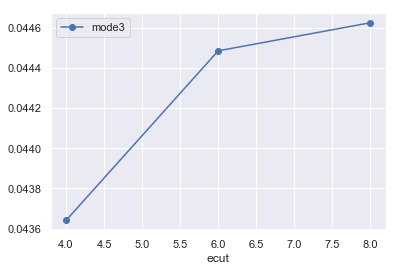Let's plot all the modes in different subplots with:

In :
data_gamma.plot(x="ecut", y=[k for k in data_gamma if k.startswith("mode")], subplots=True, style="-o");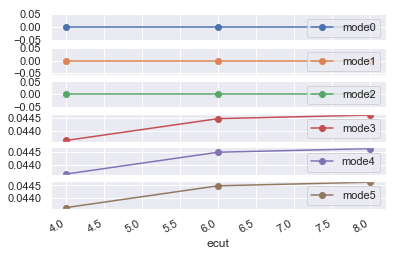This convergence study at $\Gamma$ thus reveals that our pseudos require an ecut >= 6 Ha to get reasonably converged phonon frequencies at $\Gamma$. In what follows, we assume that also the modes at the other $q$-points present a similar convergence behaviour and we use ecut = 6 Ha to keep the computational cost low.

For a quick introduction to Pandas, see:

## Phonon band structure of AlAs¶

Now we are finally ready for the calculation of the vibrational spectrum of $AlAs$. We already managed to run DFPT calculations at $\Gamma$ with different values of ecut and the steps required to get a full band structure are not that different, provided that the following differences are taken into account:

• we need the dynamical matrix $D(q)$ on a homogeneous mesh so that it is possible to calculate $D(R)$ in anaddb via Fourier transform and then phonon frequencies for arbitrary q-points via Fourier interpolation

• $AlAs$ is a polar semiconductor so we need to include the LO-TO splitting for $q \rightarrow 0$ that, in turns, requires the DFPT computation of the Born effective charges and of the dielectric constant.

In AbiPy, these concepts are translated in an easy-to-use API in which you pass an initial AbinitInput object, you specify the q-mesh for phonons in terms of ph_nqpt and activate the computation of the Born effective charges with the boolean flag with_becs.

Let's have a look at the code (as usual there are more comments than lines of code):

In :
from lesson_dfpt import build_flow_alas_phonons
abilab.print_source(build_flow_alas_phonons)

Out:
def build_flow_alas_phonons(options):
"""
Build and return a Flow to compute the dynamical matrix on a (2, 2, 2) qmesh
as well as DDK and Born effective charges.
The final DDB with all perturbations will be merged automatically and placed
in the Flow outdir directory.
"""
scf_input = make_scf_input(ecut=6, ngkpt=(4, 4, 4))
return flowtk.PhononFlow.from_scf_input("flow_alas_phonons", scf_input,
ph_ngqpt=(2, 2, 2), with_becs=True)


We can finally construct the flow with:

In :
flow_phbands = build_flow_alas_phonons(options=None)


and visualize the connections with:

In :
flow_phbands.get_graphviz()
#flow_phbands.plot_networkx();

Out:

Note that there are a lot of things happening under the hood here.

First of all, AbiPy generates PhononTasks only for the $q$-points in the irreducible wedge of the Brillouin zone corresponding to ph_ngqpt. Moreover, for a given $q$-point, only the irreducible atomic perturbations are explicitly computed since the other atomic perturbations can be reconstructed by symmetry. Fortunately you do not have to care about all these technical details as AbiPy and Abinit will automate the whole procedure.

Remember that the $q$-point mesh cannot be chosen arbitrarily since all $q$ wavevectors should connect two $k$ points of the grid used for the electrons.

It is also worth stressing that the computational cost of the DFPT run depends on the q-point since only those symmetries that preserve the q-point as well as the direction of the perturbation can be employed (calculations at $\Gamma$ are therefore much faster than other q-points).

In :
flow_phbands.get_vars_dataframe("rfphon", "rfatpol", "rfdir", "qpt", "kptopt")

Out:
rfphon rfatpol rfdir qpt kptopt class
w0_t0 None None None None None ScfTask
w1_t0 None None (1, 0, 0) (0, 0, 0) 2 DdkTask
w1_t1 None None (0, 1, 0) (0, 0, 0) 2 DdkTask
w1_t2 None None (0, 0, 1) (0, 0, 0) 2 DdkTask
w1_t3 1 [1, 1] [1, 0, 0] (0, 0, 0) 2 BecTask
w1_t4 1 [2, 2] [1, 0, 0] (0, 0, 0) 2 BecTask
w2_t0 1 [1, 1] [1, 0, 0] [0.5, 0.0, 0.0] 3 PhononTask
w2_t1 1 [1, 1] [0, 1, 0] [0.5, 0.0, 0.0] 3 PhononTask
w2_t2 1 [2, 2] [1, 0, 0] [0.5, 0.0, 0.0] 3 PhononTask
w2_t3 1 [2, 2] [0, 1, 0] [0.5, 0.0, 0.0] 3 PhononTask
w3_t0 1 [1, 1] [1, 0, 0] [0.5, 0.5, 0.0] 3 PhononTask
w3_t1 1 [2, 2] [1, 0, 0] [0.5, 0.5, 0.0] 3 PhononTask

Now we can generate the directories and the input files of the Flow. Change lesson_dfpt.py so that the build_flow_alas_phonons function is called in main instead of build_flow_alas_ecut_conv. Run the script to generate the directory with the flow. Finally, use

abirun.py flow_alas_phonons scheduler



to launch the entire calculation.

## Post-processing the results¶

Our flow is completed and we have the final DDB file with all the $q$-points and all the independent atomic perturbations. Let's open this DDB file with:

In :
ddb = abilab.abiopen("flow_alas_phonons/outdata/out_DDB")
print(ddb)

================================= File Info =================================
Name: out_DDB
Directory: /Users/gmatteo/git_repos/abitutorials/abitutorials/dfpt/flow_alas_phonons/outdata
Size: 28.11 kb
Access Time: Sun Aug 12 00:43:48 2018
Modification Time: Thu Oct 12 22:34:40 2017
Change Time: Thu Oct 12 22:34:40 2017

================================= Structure =================================
Full Formula (Al1 As1)
Reduced Formula: AlAs
abc   :   3.970101   3.970101   3.970101
angles:  60.000000  60.000000  60.000000
Sites (2)
#  SP       a     b     c
---  ----  ----  ----  ----
0  Al    0     0     0
1  As    0.25  0.25  0.25

Abinit Spacegroup: spgid: 0, num_spatial_symmetries: 24, has_timerev: True, symmorphic: False

================================== DDB Info ==================================

Number of q-points in DDB: 3
guessed_ngqpt: [2 2 2] (guess for the q-mesh divisions made by AbiPy)
Has total energy: False, Has forces: False
Has stress tensor: False

Has (at least one) atomic pertubation: True
Has (at least one diagonal) electric-field perturbation: True
Has (at least one) Born effective charge: True
Has (all) strain terms: False
Has (all) internal strain terms: False
Has (all) piezoelectric terms: False


The DdbFile object provides an easy-to-use interface that invokes anaddb to post-process the data stored in the DDB file.

anacompare_phdos, for example, computes the phonon DOS with different $q$-meshes. Each mesh is defined by a single integer, nqsmall, that gives the number of divisions used to sample the smallest reciprocal lattice vector. The number of divisions along the other directions are chosen so that proportions are preserved:

In :
c = ddb.anacompare_phdos(nqsmalls=[8, 10, 12, 14, 16])

In :
c.plotter.combiplot();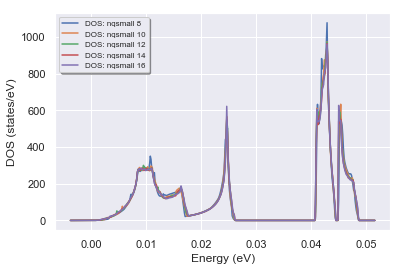A 16x16x16 $q$-mesh with the tethraedron method gives a well converged phonon DOS.

To function anaget_phbst_and_phdos_files allows one to compute the phonon band structure on an automatically defined $q$-path as well as the the phonon DOS:

In :
phbst_file, phdos_file = ddb.anaget_phbst_and_phdos_files(ndivsm=10, nqsmall=16, lo_to_splitting=False)

# Extract the phonon bands and the phonon DOS from phbst_file and phdos_file
phbands = phbst_file.phbands
phdos = phdos_file.phdos


Let's plot the bands with matplotlib:

In :
phbands.plot();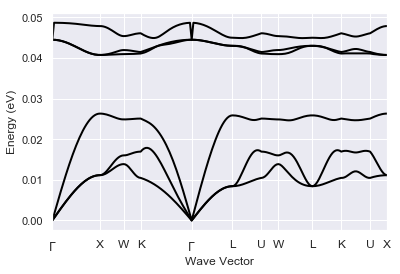and the high-symmetry q-path:

In :
phbands.qpoints.plot();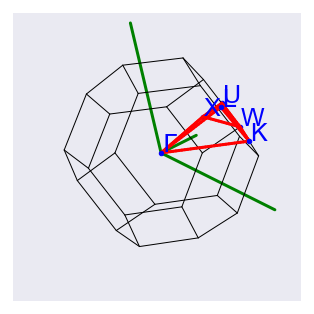Do you see the two strange dips for the highest phonon band, at the $\Gamma$ point? They are due to the lack of LO-TO splitting for the ANADDB treatment of the first list of vector. See also the discussion in the second DFPT lesson.

For years, Abinit users had to patch manually the output frequencies to include the LO-TO splitting. These days are finally gone and we can plot the LO-TO splitting with AbiPy by just setting lo_to_splitting=True:

In :
phbst_file, phdos_file = ddb.anaget_phbst_and_phdos_files(ndivsm=10, nqsmall=16, lo_to_splitting=True)

# Extract the phonon bands and the phonon DOS from phbst_file and phdos_file
phbands = phbst_file.phbands
phdos = phdos_file.phdos

In :
#phbst_file.phbands.qpoints.plot();


The band structure plot now correctly shows the non-analytical behaviour around $\Gamma$:

In :
phbands.plot();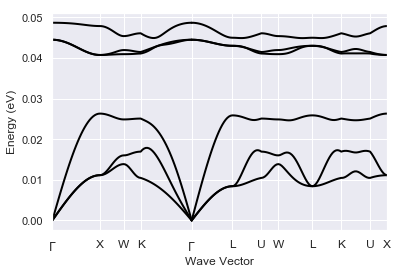lo_to_splitting=True works only when the DDB contains the Born effective charges and the dielectric constant, that must be computed in the Abinit run.

To plot bands and DOS on the same figure:

In :
phbands.plot_with_phdos(phdos);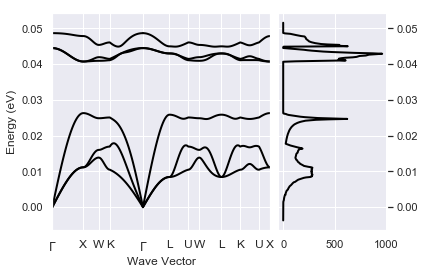The PhdosFile contains the phonon frequencies, the displacement vectors as well as the decomposition of the total DOS in terms of the contributions due to the different types of atom in the unit cell. This means that one can plot the type-projected phonon DOS with:

In :
phdos_file.plot_pjdos_type(title="AlAs type-projected phonon DOS");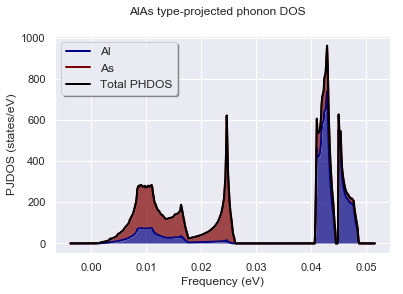and it is even possible to plot fatbands and type-projected DOSes on the same figure with:

In :
phbands.plot_fatbands(phdos_file=phdos_file);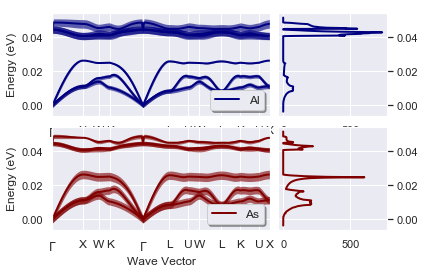The highest frequency modes have a strong Al-character while the low frequency modes originate from As. This behaviour is somehow expected. Could you explain it in terms of a simple physical model?

## Macroscopic dielectric tensor and Born effective charges¶

Our calculations includes the response of the system to an external electric field. The code below extracts the macroscopic dielectric tensor (emacro) and the Born effective charges (becs) from the DDB file:

In :
emacro, becs = ddb.anaget_emacro_and_becs()

In :
emacro

Out:
DielectricTensor([[ 1.03961652e+01 -0.00000000e+00 -0.00000000e+00]
[-0.00000000e+00  1.03961652e+01  1.19611765e-15]
[-0.00000000e+00  1.19611765e-15  1.03961652e+01]])
In :
becs

Out:
element frac_coords xx yy zz yz xz xy
0 Al [0.0, 0.0, 0.0] 2.168053 2.168053 2.168053 -2.087488e-19 -2.087488e-19 0.0
1 As [0.25, 0.25, 0.25] -2.168053 -2.168053 -2.168053 2.087488e-19 2.087488e-19 0.0

As explained in the references, the Born effective charges must fulfill the charge neutrality sum-rule. This rule is usually broken due to the discretization introduced by the FFT mesh, and anaddb will enforce it if chneut is set to 1 (default behaviour). Let's check it out!

In :
print(becs)

Born effective charges in Cartesian coordinates (Voigt notation)
element         frac_coords        xx        yy        zz            yz            xz   xy
0      Al     [0.0, 0.0, 0.0]  2.168053  2.168053  2.168053 -2.087488e-19 -2.087488e-19  0.0
1      As  [0.25, 0.25, 0.25] -2.168053 -2.168053 -2.168053  2.087488e-19  2.087488e-19  0.0

Born effective charge neutrality sum-rule with chneut: 1

[[0.00000000e+00 0.00000000e+00 0.00000000e+00]
[0.00000000e+00 0.00000000e+00 0.00000000e+00]
[2.40741243e-35 2.40741243e-35 0.00000000e+00]]


Let's repeat the same calculation but without enforcing the sum-rule:

In :
emacro, becs_chneut0 = ddb.anaget_emacro_and_becs(chneut=0)
print(becs_chneut0)

Born effective charges in Cartesian coordinates (Voigt notation)
element         frac_coords        xx        yy        zz            yz            xz   xy
0      Al     [0.0, 0.0, 0.0]  2.127295  2.127295  2.127295  8.199282e-20  8.199282e-20  0.0
1      As  [0.25, 0.25, 0.25] -2.208811 -2.208811 -2.208811  4.994904e-19  4.994904e-19  0.0

Born effective charge neutrality sum-rule with chneut: 0

[[-8.15163891e-02  0.00000000e+00  0.00000000e+00]
[ 0.00000000e+00 -8.15163891e-02  0.00000000e+00]
[ 5.81483171e-19  5.81483171e-19 -8.15163891e-02]]


## Thermodynamic properties within the harmonic approximation¶

The thermodynamic properties of an ensemble of non-interacting phonons can be expresses in terms of integrals of the DOS.

\begin{equation} %\label{eq:helmholtz} \Delta F = 3nNk_BT\int_{0}^{\omega_L}\text{ln}\left(2\text{sinh}\frac{\hbar\omega}{2k_BT}\right)g(\omega)d\omega \end{equation}\begin{equation} %\label{eq:free_en} \Delta E = 3nN\frac{\hbar}{2}\int_{0}^{\omega_L}\omega\text{coth}\left(\frac{\hbar\omega}{2k_BT}\right)g(\omega)d\omega \end{equation}\begin{equation} %\label{eq:c_v} C_v = 3nNk_B\int_{0}^{\omega_L}\left(\frac{\hbar\omega}{2k_BT}\right)^2\text{csch}^2\left(\frac{\hbar\omega}{2k_BT}\right)g(\omega)d\omega \end{equation}\begin{equation} %\label{eq:entropy} S = 3nNk_B\int_{0}^{\omega_L}\left(\frac{\hbar\omega}{2k_BT}\text{coth}\left(\frac{\hbar\omega}{2k_BT}\right) - \text{ln}\left(2\text{sinh}\frac{\hbar\omega}{2k_BT}\right)\right)g(\omega)d\omega, \end{equation}

where $k_B$ is the Boltzmann constant.

This should represent a reasonable approximation especially in the low temperature regime in which anharmonic effects can be neglected.

Let's plot the vibrational contributions thermodynamic properties as function of $T$:

In :
phdos.plot_harmonic_thermo();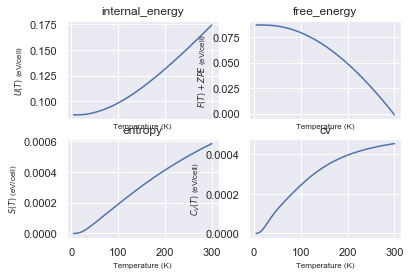## Exercises¶

• Our first phonon band structure has been computed with a (4, 4, 4) $k$-mesh for the electrons and a (2, 2, 2) $q$-mesh for phonons. You may try to increase the density of $k$-points/$q$-points to see if this change affects the final results.

• Why do you get an error from AbiPy if you try ngkpt = (4, 4, 4,) and ngqpt = (3, 3, 3)?

Back to the main Index

In [ ]:

`